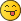Help calculating piston rod angle

RE: Help calculating piston rod angle
#6
(2019-09-26, 14:09)Philippe Hurbain Wrote: Yes, you need to do a bit of trigonometry here...

We want to turn the piston arm so that its tip is at the distance between red pin and initial axis of arm (vertical here). Since I put the gear on the origin, this distance is directly pin X coordinates (13.856), but you can also derive it in the general case from LDCad measurements as sin(16.10°)*49.96. Note that the blue parts are just helpers parts to get the right measurements points.
Once we get this value, we calculate piston arm rotation angle as arcsin(13.856/60)=13.35°. Rotate the arm by this value and use snapping on red pin to correctly position the pistion arm...

Ah, yes, that's what I was afraid of: the 't' word would come into play!OK, so, since I have to do this again a few times, let me try putting this into a sequence that makes sense to me:
• We need to know the "x" distance from A to C, which forms an opposite triangle leg to angle B.
• We also know the hypotenuse of that triangle (BC), and we know angle B, so we can use sine to solve for "x" because sine is the ratio of opposite (x) to hypotenuse (BC); thus:
• sin(B) = x / (BC) and sin(B)*(BC) = x
• Once the piston rod is rotated, it will become the hypotenuse (B'C) of a new triangle, with a known length of 60.
• x is still the opposite leg to a new angle B', which we need to find. Since we want an angle instead of a length, we use the inverse of sine, or arcsin; thus:
• arcsin( x / B'C ) = B' or arcsin( x / 60 ) = B'
• Rotate the piston rod around point B by angle B', then use part snapping to move the rotated point A onto point C (and consequently, point B onto point B').
To get angle B' using Selection Info, type the following into a calculator:
(angle B) [sin] * (distance B-C) / 60 = [sin-1] =

How'd I do?
« Next Oldest | Next Newest »

 Messages In This Thread Help calculating piston rod angle - by N. W. Perry - 2019-09-26, 3:59 RE: Help calculating piston rod angle - by David Manley - 2019-09-26, 7:34 RE: Help calculating piston rod angle - by N. W. Perry - 2019-09-26, 13:20 RE: Help calculating piston rod angle - by Philippe Hurbain - 2019-09-26, 14:09 RE: Help calculating piston rod angle - by Roland Melkert - 2019-09-26, 19:25 RE: Help calculating piston rod angle - by Philippe Hurbain - 2019-09-27, 7:13 RE: Help calculating piston rod angle - by Roland Melkert - 2019-09-27, 17:43 RE: Help calculating piston rod angle - by N. W. Perry - 2019-09-27, 5:44 RE: Help calculating piston rod angle - by Philippe Hurbain - 2019-09-27, 6:48 RE: Help calculating piston rod angle - by N. W. Perry - 2019-09-27, 13:06

Forum Jump:

Users browsing this thread: 1 Guest(s)